# Performance Analysis of an Adsorption Refrigeration System Working on Activated Carbon/Methanol Pair Using Finned Tube Type Adsorber Bed

Performance Analysis of an Adsorption Refrigeration System Working on Activated Carbon/Methanol Pair Using Finned Tube Type Adsorber Bed

Palash Soni Sruthi Lolalis Bidyut Mazumdar Shubhankar Bhowmick Vivek Kumar Gaba

Department of Mechanical Engineering, National Institute of Technology Raipur, CG 492010, India

Department of Chemical Engineering, National Institute of Technology Raipur, CG 492010, India

Corresponding Author Email:
vgaba.mech@nitrr.ac.in
Page:
1335-1342
|
DOI:
https://doi.org/10.18280/ijht.390433
4 September 2020
|
Accepted:
27 July 2021
|
Published:
31 August 2021
| Citation

OPEN ACCESS

Abstract:

Adsorption refrigeration, being a unique and eco-friendly technology, has gained popularity over conventional refrigeration systems. The present study is aimed at developing an annular finned tube adsorber model which serves as a thermal compressor in adsorption refrigeration systems. The mathematical model is addressed numerically using finite difference discretization method and explicit scheme was used for the solution. The generalized model has been simulated for activated carbon–methanol working pair. The system has an optimum cycle time of 1800s. It was found to have a highest refrigeration capacity of 260.66 kJ/kg at a regeneration temperature of 393 K and evaporator temperature of 283 K. The highest COP (Coefficient of Performance) achieved by the system is 0.3706 at a regeneration temperature of 353 K and evaporator temperature of 283 K. A highest SCP (Specific Cooling Power) of 144.8 W/kg was obtained at an evaporator temperature of 283 K and regeneration temperature of 393 K.

Keywords:

1. Introduction

The major concerns of 21st century are energy crisis, pollution control and promoting sustainable development. These considerations have influenced almost all fields of science and technology. The strict laws and regulations, in effect, also enforce the development and improvement of techniques to deal with it. The dependency on conventional energy sources must be replaced with renewable ones to initiate and maintain sustainable development.

One of the advantages of adsorption systems is that they can be powered by renewable energy like solar energy. Curran  studied solar powered cooling systems and defined coefficient of performance as a common factor for comparison. Sur et al.  presented a thermodynamic model to evaluate the performance of a single bed single stage adsorption refrigeration system working on Silica-gel/methanol pair. The solar heat collected through a flat plate collector is used as a heat source for this system. A new design of adsorber bed (Shall and tube type) is also proposed in this study. This system produced a cooling effect of 577 kJ with a COP of 0.38 corresponding to a maximum bed temperature of 90℃. Suzuki and Sakoda  conducted a basic study of solar cooling systems and reported a model to fit the experimental data which provided a first step model for estimating mass and heat transfer in adsorbent beds. The dependency of adsorption on regeneration temperature using equilibrium relations was reported.

As the literature survey indicates existence of a lot of studies on performance of activated carbon-methanol, the investigation of performance of the same on finned type adsorber/desorber bed is not available in close sight. Furthermore, with water as refrigerant, the absorption system is eco-friendly, however methanol has lower desorption temperature (below 90℃), which in with low grade energy systems is more realistic to attain as compared to desorption temperature of water which is above 100℃. Another limitation of using water as refrigerant is its freezing temperature. This is the reason behind opting activated carbon-methanol as working pair in the present study. The present study, hence addresses the performance of activated carbon-methanol adsorption system with finned adsorber/desorber bed. In the present mechno-chemical study, a numerical investigation of the performance of finned tube adsorber working on Activated carbon-methanol under different operating conditions is carried out. The present work focuses on determining the effect of evaporator temperature and regeneration temperature on the performance of the system by analyzing the parameters like COP, SCP and refrigeration effect.

2. Mathematical Formulation

## 1.pngFigure 1. Basic adsorption refrigeration cycle 

2.2 Geometry

The study is performed on a finned tube adsorber of geometry shown in the Figure 2. As shown in the figure, activated carbon is packed in the compartments formed in between two consecutive fins. The refrigerant vapour enters the adsorber through the perforated finned surface. The vapour then moves through the bed to reach the inner portions. The refrigerant is assumed to be adsorbed in vapour phase only. The adsorption in the bulk as well as pores of the particles is considered. As adsorption phenomenon is exothermic in nature, cold secondary fluid (water) is circulated through the pipes to carry away the heat of adsorption thus maintaining the temperature inside the packed bed.

Similarly, during desorption part of cycle, hot secondary fluid is circulated to release methanol to the perforated bed from activated carbon packed bed. Hence, for continuous supply of refrigerant to be maintained with in the refrigeration cycle, multiple beds can be used by introducing simultaneous adsorption and desorption part of cycle in different beds. This also leads to reduction of the idle time in the refrigeration cycle. Freundlich isotherm equation is used to model adsorption of methanol in activated carbon and the following equation was developed .

${{q}^{*}}=K{{\left( \frac{P}{{{P}_{s}}} \right)}^{{}^{1}/{}_{n}}}$      (1)

$K=\varepsilon \frac{\rho }{{{\rho }^{*}}}$       (2)

The difference in amount of adsorption before and after adsorption has been calculated using the following equation .

$\Delta q=K\left[ {{\left( \frac{{{P}_{s}}\left( {{T}_{ev}} \right)}{{{P}_{s}}\left( {{T}_{a}} \right)} \right)}^{{}^{1}/{}_{n}}}-{{\left( \frac{{{P}_{s}}\left( {{T}_{cond}} \right)}{{{P}_{s}}\left( {{T}_{reg}} \right)} \right)}^{{}^{1}/{}_{n}}} \right]$      (3)

## 2a.png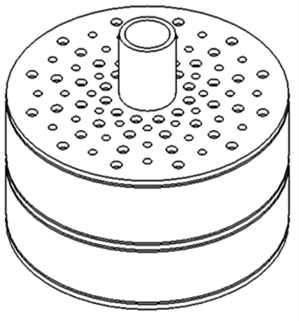## 2b.png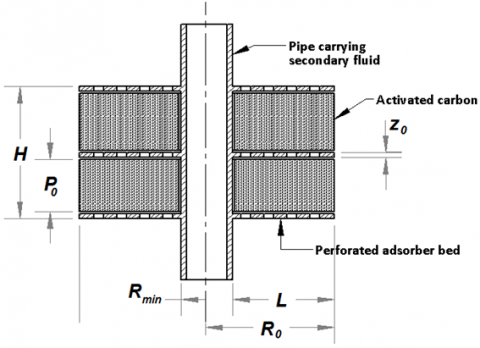Figure 2. Isometric and sectional view of finned tube adsorber

2.3 Governing equation

The governing equation is derived by considering mass transfer in particle, based on solid – diffusion model of intra particle diffusion in particles . The particles in the bed were assumed to be spherical in shape.

$\frac{\partial {{q}_{p}}}{\partial t}=D\left( \frac{{{\partial }^{2}}{{q}_{p}}}{\partial {{r}^{2}}}+\frac{2}{r}\frac{\partial {{q}_{p}}}{\partial r} \right)$      (4)

The average value of adsorption amount can be expressed using the following equation:

${{q}_{{{p}_{ave}}}}=\underset{0}{\overset{{{r}_{0}}}{\mathop \int }}\,{{r}^{2}}qdr$      (5)

The mass transfer in bed was modeled with assumptions that the particles are uniformly distributed in the bed and refrigerant is adsorbed in the vapor phase:

$\varepsilon \frac{\partial \rho_{b}}{\partial t}+(1-\varepsilon) \frac{\partial q_{p_{a v e}}}{\partial t}=\frac{K}{\mu \rho_{0}}\left[\begin{array}{l} \rho_{b}\left(\begin{array}{l} \frac{\partial^{2} \rho_{b}}{\partial R^{2}}+\frac{1}{R} \frac{\partial \rho_{b}}{\partial R} \\ +\frac{\partial^{2} \rho_{b}}{\partial Z^{2}} \end{array}\right) \\ +\left(\frac{\partial \rho_{b}}{\partial R}\right)^{2}+\left(\frac{\partial \rho_{b}}{\partial Z}\right)^{2} \end{array}\right]$      (6)

2.4 Initial conditions (at t = 0.0)

For solid diffusion model of intra particle diffusion of spherical particles:

$q_{p i}=q_{p a} \text { For } 0<\boldsymbol{r}<\boldsymbol{r}$      (7)

For mass transfer in the bed

$\rho_{b i}=\rho_{p a} \text { For } \boldsymbol{R}_{\boldsymbol{m i n}}<\boldsymbol{R}<\boldsymbol{R}_{0}, 0<\boldsymbol{Z}<\boldsymbol{Z}_{0}$      (8)

Desorption process:

For solid diffusion model of intra particle diffusion of spherical particles:

$q_{p i}=q_{p d}$ For 0 < r < r0        (9)

For mass transfer in the bed

$\rho_{b i}=\rho_{p d}$  For Rmin<R<R0, 0<Z<Z0      (10)

2.5 Boundary conditions

At r = 0;

$\frac{\partial q_{p}}{\partial r}=0$     (11)

At r = r0;

qp = qpmax       (12)

For mass transfer in the bed

At R = Rmin, 0 < Z < Z0;

$\frac{\partial \rho_{b}}{\partial R}=0$      (13)

At R = R0, 0 < Z < Z0;

$\rho_{b}=\rho_{b i}$       (14)

At Z = 0, Z0, Rmin < R < R0

$\frac{\partial \rho_{b}}{\partial Z}=0$       (15)

2.6 Solution methodology

Assuming i to be the variation along radial direction of adsorbent particle, j being the variation along radial direction of adsorber, m being the variation along axial direction of adsorber and k being the variation of time the differential terms in Eq. (4) and Eq. (6) were discretized using the finite difference method  and the following difference equations were obtained respectively:

$\frac{\partial\left(q_{p}\right)_{k}^{i}}{\partial t}=D\left[\begin{array}{l} \left(\frac{\left(q_{p}\right)_{k}^{i+1}-2\left(q_{p}\right)_{k}^{i}+\left(q_{p}\right)_{k}^{i-1}}{d R^{2}}\right) \\ +\frac{2}{R^{i}}\left(\frac{\left(q_{p}\right)_{k}^{i+1}-\left(q_{p}\right)_{k}^{i-1}}{2 d R}\right) \end{array}\right]$      (16)

$\varepsilon \frac{\partial\left(\rho_{b}\right)_{k}^{j, m}}{\partial t}+(1-\varepsilon) \frac{\partial q_{a v e}}{\partial t}=\frac{K}{\mu \rho_{0}}\left(\left(\rho_{b}\right)_{k}^{j, m} A+B\right)$      (17)

where

A=\left[ \left( \begin{align}& \frac{\left( {{\rho }_{b}} \right)_{k}^{j+1,m}-2\left( {{\rho }_{b}} \right)_{k}^{j,m}+\left( {{\rho }_{b}} \right)_{k}^{j-1,m}}{d{{R}^{2}}}+ \\& \frac{1}{{{R}^{j}}}\frac{\left( {{\rho }_{b}} \right)_{k}^{j+1,m}-\left( {{\rho }_{b}} \right)_{k}^{j-1,m}}{2dR}+ \\ & \frac{\left( {{\rho }_{b}} \right)_{k}^{j,m+1}-2\left( {{\rho }_{b}} \right)_{k}^{j,m}+\left( {{\rho }_{b}} \right)_{k}^{j,m-1}}{d{{Z}^{2}}} \\\end{align} \right) \right]B=\left[ \begin{align} & {{\left( \frac{\left( {{\rho }_{b}} \right)_{k}^{j+1,m}-\left( {{\rho }_{b}} \right)_{k}^{j-1,m}}{2dR} \right)}^{2}}+ \\ & {{\left( \frac{\left( {{\rho }_{b}} \right)_{k}^{j,m+1}-\left( {{\rho }_{b}} \right)_{k}^{j,m-1}}{2dZ} \right)}^{2}} \\\end{align} \right]

These unsteady state equations were solved using explicit scheme in the time domain .

2.7 Performance calculation of the system

COP of the system is defined as the ratio of adsorbed heat by the refrigerant in the evaporator to the net heat supplied to the system. The COP of this system is given by:

$C O P=\frac{Q_{e}}{Q_{g}}$      (18)

where, $Q_{e}$  is the heat adsorbed by the refrigerant from the evaporator and $Q_{g}$  is the net heat supplied to the system during desorption process and is given by as follows.

${{Q}_{e}}={{m}_{rg}}\left( {{h}_{1}}-{{h}_{3}} \right)$

where, $h_{1} \text { and } h_{3}$ are the enthalpy of refrigerant methanol at the exit and inlet of evaporator respectively.

$m_{r g}$  is the total mass of refrigerant methanol flow through the evaporator and is given by:

${{m}_{rg}}={{m}_{a}}\times \Delta q$

and

{{Q}_{g}}=\left[ \begin{align} & {{m}_{a}}{{C}_{pa}}d{{T}_{a}}+\left( {{m}_{ri}}-{{m}_{rg}} \right){{C}_{pr}}d{{T}_{r}}+ \\ & {{m}_{rg}}\left( {{h}_{2}}-{{h}_{1}} \right)+{{m}_{m}}{{C}_{pm}}d{{T}_{m}}+{{m}_{rg}}{{h}_{sg}} \\\end{align} \right]

where, $h_{2} \text { and } h_{1}$ are the enthalpy of refrigerant methanol at the exit and inlet of adsorber bed respectively.

Specific cooling power (W/kg) is define as the cooling effect produced per kg mass of the adsorbent.

$S C P=\frac{Q_{e}}{M_{\text {ads }}\quad t_{\text {cycle }}}$       (19)

mads is the mass of adsorbent (Activated carbon) in kg and tcycleis the total cycle time of the system.

Refrigeration effect (kJ/kg):

$R E=\frac{Q_{e}}{M_{a d s}}$     (20)

3. Results and Discussions

In the present work, the amount adsorbed and regenerated from the bed was calculated for different regeneration temperatures and evaporator temperatures. The regeneration temperature was varied from 353 K to 393 K while the evaporator temperature was varied from 268 K to 283 K. The study is limited to 393 K regeneration temperature as the system is designed to utilize low grade energy such solar and waste heat sources and achieving a temperature beyond 393 K is difficult.

The numerical values of system geometries and properties are L= 0.81 m, H=0.4 m, P0=0.175 m, Z0=0.025 m, R0 = 0.9 m, ρ* = 360 kg/m3, ϵp = 0.6 and ρp =271.5 kg/m3.

The theoretical model, thus developed into a computational code, is validated with benchmark results  for silica gel–water adsorption refrigeration system. It was observed that the results from the proposed model showed strong agreement with those from reference with an error of within 5% as plotted in Figure 3. Hence the model can be considered valid for an adsorption refrigeration system working with the same principle, that is, physical adsorption.

In Figure 4 the capacity of adsorption of methanol (∆q) is plotted against parameter n as given in equation 3 for different regeneration temperature. It is observed that there exists an optimum value of n for each regeneration temperature at which the capacity of adsorption of methanol reaches the maximum. The plot of Δq against n shown in Figure 4 yields an optimum n value for activated carbon- methanol. It is also observed that as regeneration temperature is increased, ∆q also increases due to the increase in saturation pressure corresponding to each regeneration temperature.

## 3.png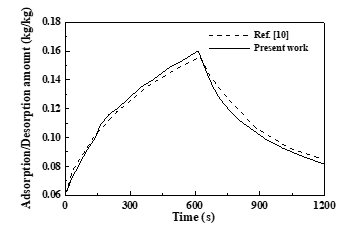Figure 3. Validation of the proposed model

## 4.png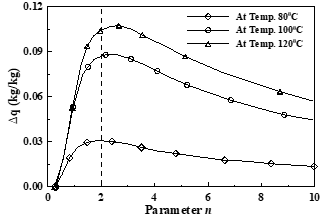Figure 4. Variation of Δq with parameter n

## 5.png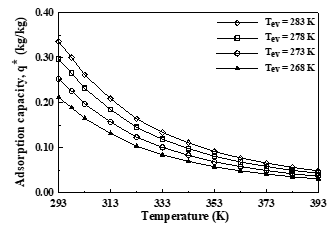Figure 5. Variation of adsorption capacity (q*) with temperature

## 6.png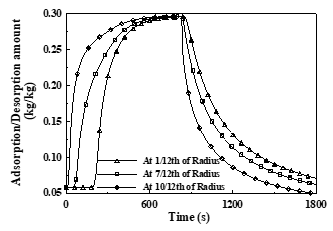Figure 6. Adsorption/desorption along radius at Treg = 393K, Tev = 283K

It is also evident that the time of adsorption and desorption are almost equal over a total span of optimum cycle. The reason may be attributed to the fact that the amount being evaporated and hence being adsorbed and getting regenerated increases with increase in evaporator temperature. However, the evaporator temperature cannot be arbitrarily increased to increase the performance as it depends on the temperature to be maintained in the desired space to be cooled. In Figure 7, the dependence of COP on evaporator temperature is plotted. The COP of proposed system is observed to increase with increase in evaporator temperature.

## 7.png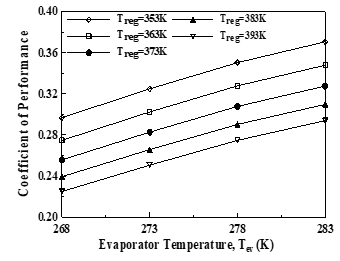Figure 7. Variation of COP with evaporator temperature

## 8.pngFigure 8. Variation of COP with regeneration temperature

In Figure 8, the variation of COP with regeneration temperature is plotted. The COP of the proposed system is reported to decrease with increase in regeneration temperature since the heat input needed to attain a particular regeneration temperature increases with increase in regeneration temperature. The COP is a measure of performance which depends on the amount of heat input needed to produce a particular refrigeration effect. Hence the COP, which is inversely proportional to heat input, decreases with increase in regeneration temperature.

The variation of SCP with regeneration temperature is shown in Figure 9. It is observed to increase with increase in regeneration temperature but the variation was not much significant which shows that regeneration temperature has less effect on the SCP of this system.

In Figure 10, the refrigeration effect is plotted against evaporator temperature. It is observed that the variation of refrigeration effect is more significant with evaporator temperature.

## 9.pngFigure 9. Variation of SCP with regeneration temperature

## 10.png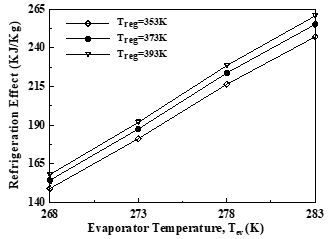Figure 10. Variation of Refrigeration effect with evaporator temperature

4. Conclusion

The adsorption amount depends on evaporator temperature and adsorbent bed temperature while the amount of regeneration depends on regeneration temperature. A maximum adsorption capacity of 0.2959 kg/kg was observed at an evaporator temperature of 283 K and regeneration temperature of 353 K. COP of an adsorption refrigeration system has inverse relation with regeneration temperature if the heat source is not kept constant. It was also found that the COP increases with evaporator temperature but it cannot be increased arbitrarily to increase the performance as it depends on the temperature to be maintained in the desired space to be cooled. Normally for cold storage, an evaporator temperature of -5℃ to 5℃ is preferred depending on the food to be stored and for air conditioning a temperature of 10℃ is preferred. A highest COP of 0.3706 was obtained at a regeneration temperature of 353 K and evaporator temperature of 283 K. The SCP of the proposed system is found to increase with increase in regeneration temperature but the variation is not much significant which shows that regeneration temperature has less effect on the SCP of proposed system. It is also observed that the variation of SCP is more significant with the evaporator temperature. It increases with increase in evaporator temperature. The highest SCP of 144.8 W/kg was obtained at a regeneration temperature of 393 K and evaporator temperature of 283 K. Refrigerating effect was also found to increase with both evaporator temperature and regeneration temperature and showed a maximum of 260.665 kJ/kg at a regeneration temperature of 393 K and evaporator temperature of 283 K. The proposed system of activated carbon-methanol working pair is, thus, reported to be well suited for air conditioning system where the evaporator temperature is around 283 K.

Acknowledgment

The authors gratefully acknowledge the support of the Department of Mechanical Engineering, Faculty of Mechanical and Chemical Engineering, National institute of technology Raipur, India.

Nomenclature
References

 Sah, R.P., Choudhury, B., Das, R.K., Sur, A. (2017). An overview of modelling techniques employed for performance simulation of low-grade heat operated adsorption cooling systems. Renewable and Sustainable Energy Reviews, 74: 364-376. https://doi.org/10.1016/j.rser.2017.02.062

 Faizan, S., Sultan, M., Miyazaki, T., Saha, B.B. (2020). Recent updates on the adsorption capacities of adsorbent-adsorbate pairs for heat transformation applications. Renewable and Sustainable Energy Reviews, 119: 109630. https://doi.org/10.1016/j.rser.2019.109630

 Soni, P., Gaba, V.K. (2019). Comparative analysis of silica-gel/water and zeolite/water pair on adsorption refrigeration system. Journal of Physics: Conference Series, 1240: 012067. https://doi:10.1088/1742-6596/1240/1/012067

 Hu, Y.H., Ruckenstein, E. (2006). Applicability of Dubinin–Astakhov equation to CO2 adsorption on single-walled carbon nanotubes. Chemical Physics Letters, 425(4-6): 306-310. https://doi.org/10.1016/j.cplett.2006.05.059

 Curran, H.M. (1977). Coefficient of performance for solar-powered space cooling systems. Solar Energy, 19(5): 601-603. https://doi.org/10.1016/0038-092X(77)90121-9

 Sur, A., Pandya, S., Sah, R.P., Kotecha, K., Narkhede, S. (2020). Influence of bed temperature on performance of silica gel/methanol adsorption refrigeration system at adsorption equilibrium. Particulate Science and Technology, 1-8. https://doi.org/10.1080/02726351.2020.1778145

 Suzuki, M., Sakoda, A. (1983). Fundamental study on solar powered adsorption cooling system. Journal of Chemical Engineering of Japan, 17(1): 52-57. https://doi.org/10.1252/jcej.17.52

 Wang, Q., Gao, X., Xu, J.Y., Maiga, A.S., Chen, G.M. (2012). Experimental investigation on a fluidized-bed adsorber/desorber for the adsorption refrigeration system. International Journal of Refrigeration, 35(3): 694-700. https://doi.org/10.1016/j.ijrefrig.2011.05.020

 Zhao, Y., Hu, E., Blazewicz, A. (2012). Dynamic modelling of an activated carbon–methanol adsorption refrigeration tube with considerations of interfacial convection and transient pressure process. Applied Energy, 95: 276-284. https://doi.org/10.1016/j.apenergy.2012.02.050

 Ji, X., Li, M., Fan, J., Zhang, P., Luo, B., Wang, L. (2014). Structure optimization and performance experiments of a solar-powered finned-tube adsorption refrigeration system. Applied Energy, 113: 1293-1300. https://doi.org/10.1016/j.apenergy.2013.08.088

 Li, L., Kubota, M., Watanabe, F., Kobayashi, N., Hasatani, M. (2004). Optimum design of a fin-type silica gel tube module in the silica gel/water adsorption heat pump. Journal of Chemical Engineering of Japan, 37(4): 551-557. https://doi.org/10.1252/jcej.37.551

 Hong, S.W., Ahn, S.H., Kwon, O.K., Chung, J.D. (2015). Optimization of a fin-tube type adsorption by design of experiment. International Journal of Refrigeration, 49: 49-56. https://doi.org/10.1016/j.ijrefrig.2014.09.022

 Liu, Y., Leong, K.C. (2006). Numerical study of a novel cascading adsorption cycle. International Journal of Refrigeration, 29(2): 250-259. https://doi.org/10.1016/j.ijrefrig.2005.05.008

 Leong, K.C., Liu, Y. (2004). Numerical modeling of combined heat and mass transfer in the adsorbent bed of a zeolite/water cooling system. Applied Thermal Engineering, 24(16): 2359-2374. https://doi.org/10.1016/j.applthermaleng.2004.02.014

 Marlinda, A., Uyun, S., Miyazaki, T., Ueda, Y., Akisawa, A. (2010). Performance analysis of a double-effect adsorption refrigeration cycle with a silica gel/water working pair. Energies, 3(11): 1704-1720. https://doi.org/10.3390/en3111704

 Deshmukh, H., Maiya, M.P., Murthy, S.S. (2015). Continuous vapour adsorption cooling system with three adsorber beds. Applied Thermal Engineering, 82: 380-389. https://doi.org/10.1016/j.applthermaleng.2015.01.013

 Sur, A., Das, R.K. (2017). Experimental investigation on waste heat driven activated carbon‑methanol adsorption cooling system. Journal of the Brazilian Society of Mechanical Sciences and Engineering, 39(7): 2735-2746. https://doi.org/10.1007/s40430-017-0792-y

 Chekirou, W., Boukheit, N., Kerbache, T. (2007). Numerical modelling of combined heat and mass transfer in a tubular adsorber of a solid adsorption solar refrigerator. Revue des Energies Renouvelables, 10(3): 367-379.

 Abd-Elhady, M.M., Hamed, A.M. (2020). Effect of fin design parameters on the performance of a two-bed adsorption chiller. International Journal of Refrigeration, 113: 164-173. https://doi.org/10.1016/j.ijrefrig.2020.01.006

 Sur, A., Sah, R.P., Pandya, S. (2020). Milk storage system for remote areas using solar thermal energy and adsorption cooling. Materials Today: Proceedings, 28(3): 1764-1770. https://doi.org/10.1016/j.matpr.2020.05.170

 Zhao, C., Wang, Y., Li, M., Zhao, W. (2020). Experimental study of a solar adsorption refrigeration system integratedwith a compound parabolic concentrator based on an enhanced masstransfer cycle in Kunming, China. Solar Energy, 195: 37-46. https://doi.org/10.1016/j.solener.2019.11.056

 Alelyani, S.M., Bertrand, W.K., Zhang, Z., Phelan, P.E. (2020). Experimental study of an evacuated tube solar adsorption cooling module and its optimal adsorbent bed design. Solar Energy, 211: 183-191. https://doi.org/10.1016/j.solener.2020.09.044

  Chihara, K., Suzuki, M. (1983). Air drying by pressure swing adsorption. Journal of Chemical Engineering of Japan, 16(4): 293-299. https://doi.org/10.1252/jcej.16.293

 Patankar, S.V. (1998). Numerical Heat Transfer and Fluid Flow. Hemisphere Publishing Corporation, Washington-New York-London. McGraw Hill Book Company, New York. https://doi.org/10.1002/cite.330530323